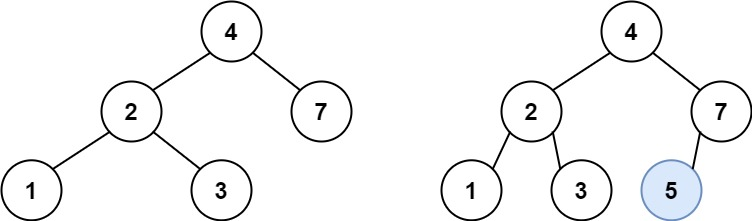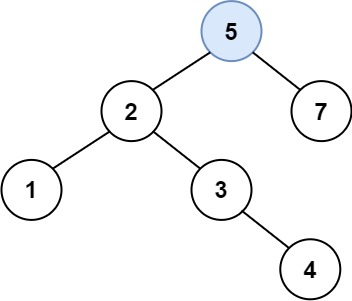## 701. Insert into a Binary Search Tree

You are given the `root` node of a binary search tree (BST) and a `value` to insert into the tree. Return the root node of the BST after the insertion. It is guaranteed that the new value does not exist in the original BST.

Notice that there may exist multiple valid ways for the insertion, as long as the tree remains a BST after insertion. You can return any of them.

Example 1:```Input: root = [4,2,7,1,3], val = 5
Output: [4,2,7,1,3,5]
Explanation: Another accepted tree is:```

Example 2:

```Input: root = [40,20,60,10,30,50,70], val = 25
Output: [40,20,60,10,30,50,70,null,null,25]
```

Example 3:

```Input: root = [4,2,7,1,3,null,null,null,null,null,null], val = 5
Output: [4,2,7,1,3,5]
```

Constraints:

• The number of nodes in the tree will be in the range `[0, 104]`.
• `-108 <= Node.val <= 108`
• All the values `Node.val` are unique.
• `-108 <= val <= 108`
• It's guaranteed that `val` does not exist in the original BST.

## Rust Solution

``````struct Solution;
use rustgym_util::*;

trait Postorder {
fn insert(self, val: i32) -> Self;
}

impl Postorder for TreeLink {
fn insert(self, val: i32) -> Self {
if let Some(node) = self {
let node_val = node.borrow().val;
let left = node.borrow_mut().left.take();
let right = node.borrow_mut().right.take();
if node_val < val {
tree!(node_val, left, right.insert(val))
} else {
tree!(node_val, left.insert(val), right)
}
} else {
tree!(val)
}
}
}

impl Solution {
fn insert_into_bst(root: TreeLink, val: i32) -> TreeLink {
root.insert(val)
}
}

#[test]
fn test() {
let root = tree!(4, tree!(2, tree!(1), tree!(3)), tree!(7));
let val = 5;
let res = tree!(4, tree!(2, tree!(1), tree!(3)), tree!(7, tree!(5), None));
assert_eq!(Solution::insert_into_bst(root, val), res);
}
``````

Having problems with this solution? Click here to submit an issue on github.